## Specifying Joint Actuation Inputs

### Actuation Modes

Joint blocks provide two actuation parameters. These parameters, Force/Torque and Motion, govern how the joint behaves during simulation. Depending on the parameter settings you select, a joint block can accept either actuation parameter as input or automatically compute its value during simulation.

An additional setting (`None`) allows you to set actuation force/torque directly to zero. The joint primitive is free to move during simulation, but it has no actuator input. Motion is due indirectly to forces and torques acting elsewhere in the model, or directly to velocity state targets.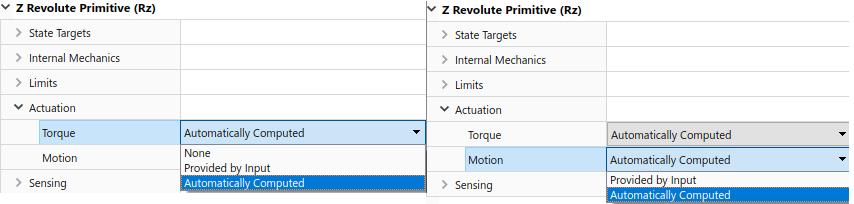Like all joint block parameters, you select the actuation parameter settings for each joint primitive separately. Different joint primitives in the same block need not share the same actuation settings. Using a Pin Slot Joint block, for example, you can provide motion input and have actuation torque automatically computed for the Z Revolute Primitive (Rz), while having motion automatically computed with no actuation force for the X Prismatic Primitive (Px).By combining different Force/Torque and Motion actuation settings, you can achieve different joint actuation modes. Forward dynamics and inverse dynamics modes are two common examples. You actuate a joint primitive in forward dynamics mode by providing actuation force/torque as input while having motion automatically computed. Conversely, you actuate a joint primitive in inverse dynamics mode by providing motion as input while having actuation force/torque automatically computed.

Other joint actuation modes, including fully computed and fully specified modes, are possible. The table summarizes the different actuation modes that you can obtain by manipulating the actuation parameter settings.

Joint Actuation Modes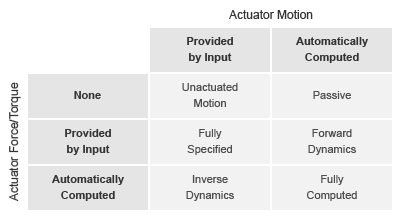More generally, thinking of joint actuation in terms of the specified or calculated quantities—i.e., force/torque and motion—provides a more practical modeling approach. You may not always know the appropriate mode for a joint but, having planned the model beforehand, you should always know the answers to two questions:

• Is the joint primitive mechanically actuated?

• Is the desired trajectory of the joint primitive known?

By selecting the joint actuation settings based on the answers to these questions, you can ensure that each joint is properly set for your application. The figure shows the proper settings depending on your answers.

Selecting Joint Primitive Actuation Settings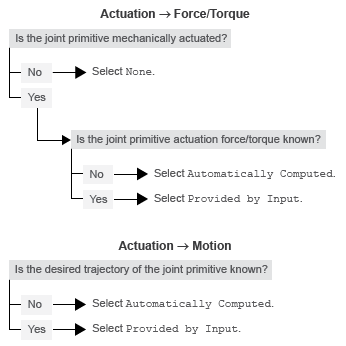### Motion Input

The motion input of a joint primitive is a timeseries object specifying that primitive’s trajectory. For a prismatic primitive, that trajectory is the position coordinate along the primitive axis, given as a function of time. The coordinate provides the position of the follower frame origin with respect to the base frame origin. The primitive axis is resolved in the base frame.

For a revolute primitive, the trajectory is the angle about the primitive axis, given as a function of time. This angle provides the rotation of the follower frame with respect to the base frame about the primitive axis. The axis is resolved in the base frame.

Spherical joint primitives provide no motion actuation options. You can specify actuation torque for these primitives, but you cannot prescribe their trajectories. Those trajectories are always automatically computed during simulation from the multibody dynamics model. For more information about multibody dynamics simulations, see Multibody Modeling.

#### Zero Motion Prescription

Unlike Actuation > Force/Torque, the Actuation > Motion parameter provides no zero input option, corresponding to a fixed joint primitive during simulation. You can, however, prescribe zero motion the same way you prescribe all other types of motion: using Simscape™ and Simulink® blocks.

In Simscape Multibody™, motion input signals are position-centric. You specify the joint primitive position and, if filtered to the second-order, the Simulink-PS Converter block smooths the signal while providing its two time-derivatives automatically. This behavior makes zero motion prescription straightforward: just provide a constant signal to the motion actuation input port of the joint primitive and simulate.

The figure shows an example of zero-motion prescription. A Simulink Constant block provides a constant position value. A Simulink-PS Converter block converts this Simulink signal into a Simscape signal compatible with the motion actuation input port of the Base-Crank Revolute Joint block. Assuming that assembly and simulation are successful, this joint will maintain a fixed angle of 30 degrees, corresponding to the value set in the Simulink Constant block and the units set in the Simulink-PS Converter block.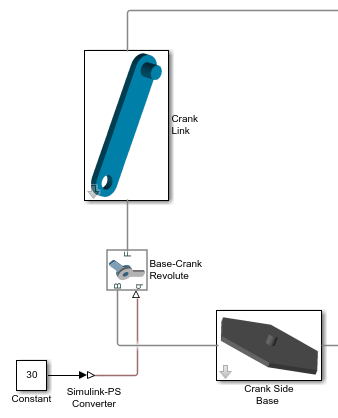### Input Handling

When prescribing a joint primitive trajectory, it is practical to specify a single input, the position, and filter that input using a Simulink-PS Converter block. This filter, which must be second-order, automatically provides the two time derivatives of the motion input. Because it also smooths the input signal, the filter can help prevent simulation issues due to sudden changes or discontinuities, such as those present when using a Simulink Step block.

Filtering smooths the input signal over a time scale of the order of the input filtering time constant. The larger the time constant, the greater the signal smoothing, and the more distorted the signal tends to become. The smaller the time constant, the closer the filtered signal is to the input signal, but also the greater the model stiffness—and, hence, the slower the simulation.

As a guideline, the input filtering time constant should be only as small as the smallest relevant time scale in a model. By default, its value is 0.001 s. While appropriate for many models, this value is often too small for Simscape Multibody models. For faster simulation, start with a value of 0.01 s. Decrease this value for greater accuracy.

If you know the two time derivatives of the motion input signal, you can specify them directly. This approach is most convenient for simple trajectories with simple derivatives. You must, however, ensure that the two derivative signals are compatible with the position signal. If they are not, even when simulation proceeds, results may be inaccurate.

### Assembly and Simulation

Simscape Multibody joints with motion inputs start simulation (Ctrl+T) at the initial position dictated by the input signal. This initial position may differ from the assembled state, which is governed by an assembly algorithm optimized to meet the joint state targets, if any. Even in the absence of joint state targets, the assembled state may differ from that at simulation time zero.

Note

You obtain the assembled state each time you update the block diagram, e.g., by pressing Ctrl+D. You obtain the initial simulation state each time you run the simulation, e.g., by pressing Ctrl+T, and pausing at time zero.

Due to the discrepancy between the two states, Model Report provides accurate initial state data only for models lacking motion inputs. For models possessing motion inputs, that data is accurate only when the initial position prescribed by the motion input signal exactly matches the initial position prescribed in the joint state targets.

Similarly, Mechanics Explorer displays the initial joint states accurately only for models lacking motion inputs. As it transitions from the assembled state to the initial simulation state, Mechanics Explorer may show a sudden jump if a model contains motion inputs that are incompatible with the joint state targets. You can eliminate the sudden change by making the initial position prescribed by joint motion inputs equal to the initial position prescribed by the joint state targets.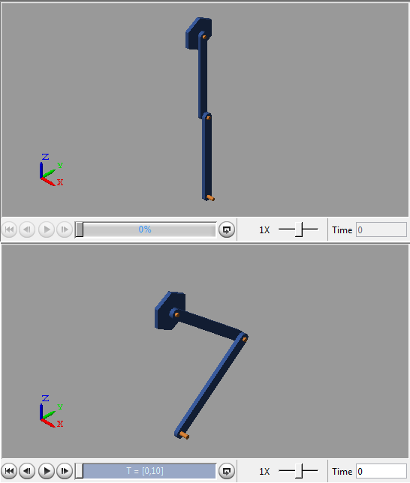### Specifying Motion Input Derivatives

If filtering the input signal using the Simulink-PS Converter block, you need only to provide the position signal. The block automatically computes the derivatives. You must, however, select second-order filtering in the block dialog box:

1. Open the dialog box of the Simulink-PS Converter block and click Input Handling.

2. In Filtering and derivatives, select `Filter input`.

3. In Input filtering order, select `Second-order filtering`.

4. In Input filtering time constant (in seconds), enter the characteristic time over which filter smooths the signal. A good starting value is `0.01` seconds.

If providing the input derivatives directly, you must first compute those derivatives. Then, using the Simulink-PS Converter block, you can provide them to the target joint block. To specify the input derivatives directly:

1. Open the Simulink-PS Converter block receiving the input signal and click the Input Handling tab.

2. In Filtering and derivatives, select `Provide input derivative(s)`.

3. To specify both derivatives, in Input derivatives, select `Provide first and second derivatives`.

The block displays two additional physical signal ports, one for each derivative.